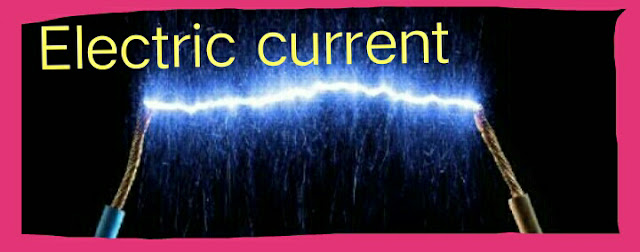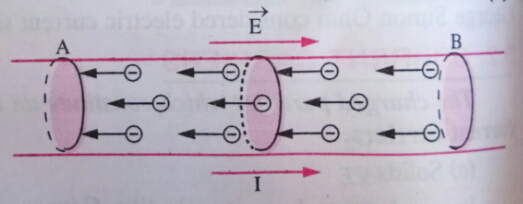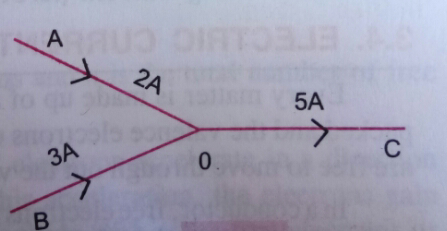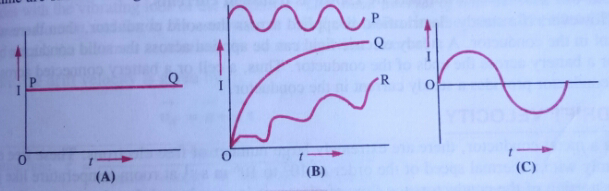Views

# Introduction :

Electric current  is defined as the rate of flow of electric charge through cross section of a conductor.  In electrostatics, we have studied the charges at rest. As the term electrostatics itself indicates. However charges in motion constitute an electric current . This effect may prove fatal or sometimes disastrous.

We know that the flow of charges in lightning is of short duration and not steady. The short duration flow of current constitute transient current. Such type of currents stop as soon as the flow of charges stops. Number of devices like a torch and a cell-driven clock in which flow of charges is steady and constitute steady Electric current . Flow of charges in a conductor  can be compared to the flow of water in a river. German physicist, George Simon Ohm considered electric current similar to heat flow in a conductor.

## Electric current :

Let us suppose charge Q crosses through a cross section of a Conductor in time t,  thenElectric current

Electric current ,  I = Total charge flowing /time taken (t)

or  I = Q/t .............(1)

If n electrons,  each of charge e ( = 1.6 × 10  ̄¹⁹  ) cross through a cross section of a conductor in time t,  then using Q = ne,  we get;

I = Q/t   =  ne/t ...................(2)

### Explanation :

An example similar to the flow of water in a river can be considered for understanding the concept of Electric current . The strength of the flow of water at any point of the river can be assessed by allowing the water to flow from a ring held in a direction perpendicular to the flow of river at that point. The idea regarding the water current is given by the amount of water flowing through the ring per second.

Now consider the portion AB of the conductor shown in the figure of uniform cross section area. This portion of the conductor is placed under the influence of an electric field (E)  applied in the direction from A to B. Now free electrons of the conductor will move from end B to end A of the conductor,  constituting electric current which is equal to the amount of charge flowing through the cross section per unit time.Electric current explanation

If the net charge ∆Q crosses the shaded cross sectional area in a time ∆t , then the instantaneous current across the conductor is given by :

I(t)        ≅    lim        ∆Q/∆t  = dQ/dt
∆t ⇒0

#### Nature  :

Electric current  is not a vector quantity. It is a scalar quantity. Although Electric current  has magnitude as well as direction yet it is not a vector quantity because it doesn't obey vector laws and the laws of simple algebra are used to find the net electric currents.Electric current Nature

For example ; Consider three metallic wires A, B and C meeting at a point or junction O (Figure). Let 2A and 3A current flows through the wires A and B respectively meeting at a point O with the third wire C. Then the current in the third wire C is 5A (=2A+3A). Thus , electric currents are added algebraically.

#### Note:

It should be remembered that the current flowing through a conductor is represented by an arrow. This arrow may not be understood as a vector. It simply represents the sense of direction of the flow of charges in a conductor.

#### Direction :

The direction of Electric current  is given by the direction of flow of positive charges. This current is know as conventional current. The reason of calling it a conventional current is that in the beginning it was thought that the electric current is due to the flow of positive charges (a convention).

As far as the conductor is concerned, the flow of electrons that is negative charge constitute Electric current  known as electronic current. The direction of electronic current is opposite to the direction of conventional current. Therefore, the direction of conventional current is opposite to the direction of electronic current.

#### Note:

Remember,  in case of a discharge tube, current carriers are electrons and hydrogen ions that is proton. Therefore, total current through a given cross section of discharge tube ,

I = (ne +pe)e/t.

#### Types of current :

Three types of Electric currents are discussed below :-

I) Alternating current : An Electric current  is said to be alternating current if its magnitude changes with time and polarity ( that is + or - ) reverses periodically that is after regular intervals of time.Types of Electric current

In case of Sinusoidal AC that is alternating current following a sine wave, the alternations of current with time are shown in figure c.

II) Steady Direct current : If the magnitude and direction of an Electric current do not change with time then it is said to be steady ( that is constant or regular)  direct current.

iii)  Variable or varying direct current : An Electric current  is said to be changing that is varying direct current if its magnitude changes with time and polarity remains same.

The variation of variable current (I)  with time (t) is shown by three different curves P, Q and R in figure B.

Thus, we can say that the current which reverses its polarity periodically is called an alternating current (A. C) , whereas the current which does not reverse its polarity is called Direct current (D. C). For example  steady current and varying current of same polarity.

#### Units :

The standard international that is S. I unit of current is Ampere (A).

one ampere = 1C S ̄¹

Definition of Ampere : Current through a conductor is said to be one ampere if one coulomb charge flows through any cross section of the conductor in one second.

#### Commonly used units are :

milliAmpere ( 1mA  = 10 ̄³ A)
microAmpere ( 1μA = 10 ̄⁶ A)
nanoAmpere (1nA = 10 ̄⁹A)
picoAmpere  (1pA = 10 ̄¹²A).

#### Note:

I)  The average current during lightning is of the order of tens of thousands of amperes.

II)  In human nerves, currents are of the order of microamperes.

iii)  Electric current  more than 0.015 A passing through a body is fatal.

Numerical illustration : Calculate the quantity of amount of current flowing through a conductor if 10⁶ electrons crosses through the cross section of the conductor in 1.6 milli second . Given , e = 1.6 × 10 ̄¹⁹ C.

Solution. Here,    n  = 10⁶ , e = 1.6 × 10 ̄¹⁹ C , t = 1.6 m    , s = 1.6 × 10 ̄³ S.

using ,          I   =  Q/t  = ne /t , we get

I =  10⁶ × 1.6 × 10 ̄¹⁹ ∕ 1.6 × 10 ̄³

=   1.0 × 10 ̄¹⁰A

#### Current carriers:

These are the charged particles which constitute an Electric current  in solids, liquids or gases.

a)  Liquids :  Some liquids which allow the electric current to pass through them and consequently dissociate into ions are called electrolytes.  For example;  CuSO4 solution , AgNO3 solution,  H2SO4 etc. These electrolytes dissociate into negative and positive ions . These ions under the influence of external electric field move in definite directions to constitute electric current. Thus positive and negative ions are responsible for carrying current in liquids.

b)  Solids : In conductors , the electrons in the outermost orbits of the atoms that is valence electrons are loosely bound. These electrons can move about in the whole conductor and are known as free electrons. These free electrons under the influence of external electric field move in a direction opposite to the direction of electric field and constitute electric current. Thus , free electrons or valence electrons are the current carriers in conductors.

c) Gases : Generally, gases behave as insulators of electricity but they get ionized and became conductors at low pressure,  when high potential difference is applied across them. Ionized gases contain positive ions and electrons. These positive ions and electrons are the current carriers in gases.

#### Conclusion:

In conclusion, Electric current  is the flow of electric charge. The article has discussed about the different topics related to electric current . It has discussed about the charge carriers, units,  direction and nature.